Altitude and Median of a triangle

Chapter 6 Class 7 Triangle and its Properties
Concept wise

Line joining vertex to mid point of opposite side of a triangle is median of a triangle.

For ∆ABC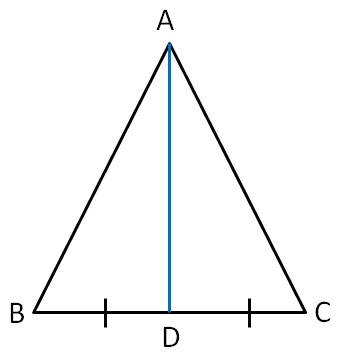Here, AD is the median of triangle ∆ABC

& D is mid-point of BC.

i.e. BD = CD

Similarly, we can draw median from point B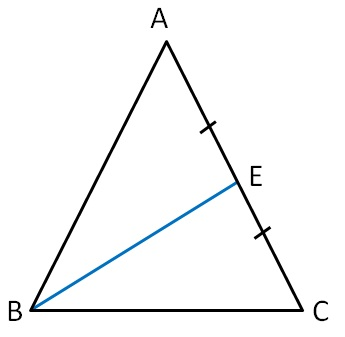Here, BE is the median of ∆ABC

& E is the mid point of AC

i.e. AE = EC.

And, we can draw median from point C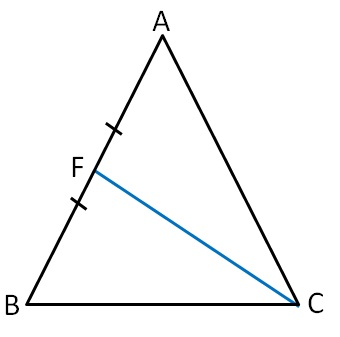Here, CF is the median of ∆ABC

& F is the mid point of AB.

i.e. AF = FB.

So, our median can be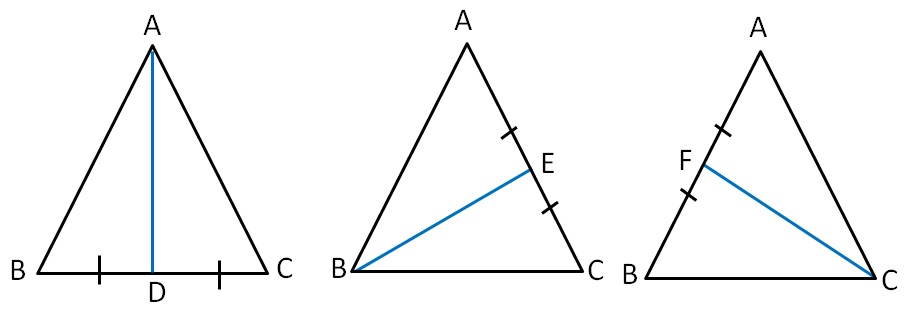## For obtuse angled triangle ∆ABC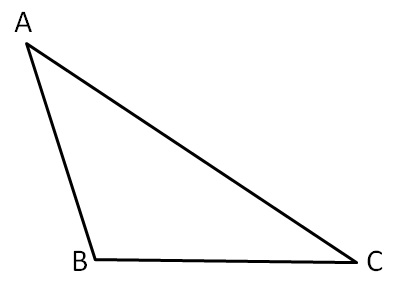Medians are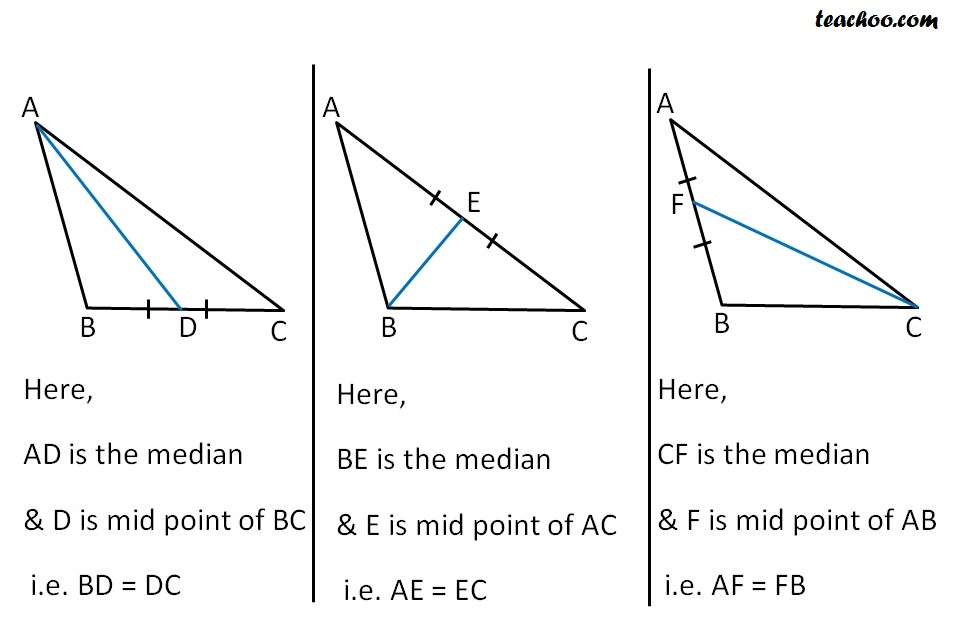### For right angled triangle ∆ABC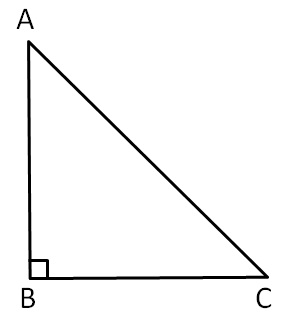Medians are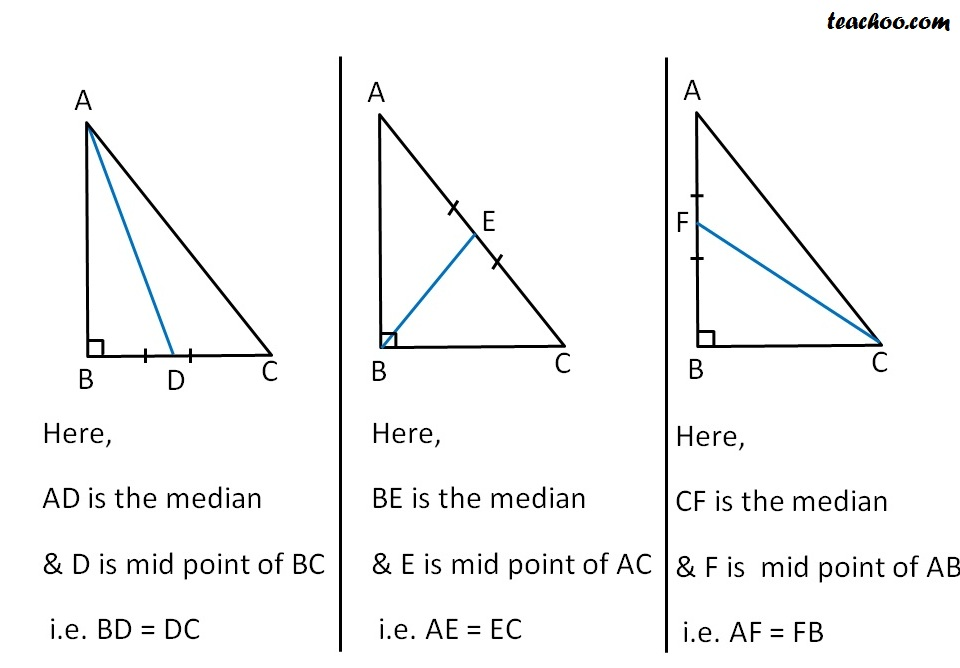Learn in your speed, with individual attention - Teachoo Maths 1-on-1 Class